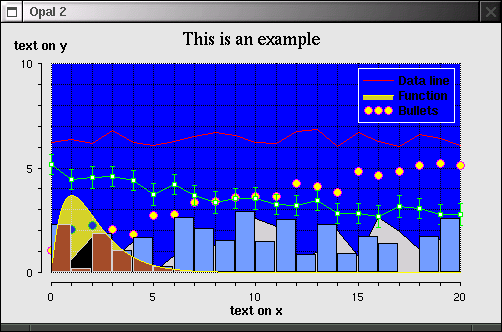# PLOT-2D FOR GARNET (COMMON LISP)

Plot-2D is a data representation package for Garnet (Common Lisp GUI Objects Library). If you use Common Lisp for scientific calculations, then Plot-2D will allow you quickly visualise your results. The great thing about Garnet is that it provides a function to output any window into a nice PostScript file, which you can later attach into your paper.The package contains the Plot-2D:plot gadget (object prototype or class) for creating 2-dimensional plots, and several objects for representation of datasets and mathematical functions. The easiest way to build plots is by using function Plot-2D:build, and function Plot-2D:update can be used to update the graphs. These functions can take as arguments either data sets or functions. The data can be in a form of list, a list of lists, or 2 dimensional array (each row is treated as a separate data set). Functions should be in a form (lambda (x) ...) and sometimes require the definition of their domains (also in a form of (lambda (x) ...), but returning T or NIL for testing). These objects are plotted using one or several data objects onto one or several plots. All parameters are handled automatically, or can be specified in the arguments of the Plot-2D:build function. Everything is customisable: colours, fonts, grid-lines, ticks on the axis, etc.

Each data set or function can have a description string that if not NIL is used automatically shown in the legend (description box) on the plot. You can drag and drop the legend box to any position on the plot with the mouse.

## Requirements

You will need Garnet GUI library for Common Lisp (it is free). Garnet provides an easy access to X-Window or Macintosh operating systems graphical interfaces. Garnet itself requires Common Lisp installed. Most of the implementations on Unix / Linux and Macintosh OS should work fine, for example CMU Common Lisp (free). Common Lisp exists probably on all platforms (PC, SGI, Sun, HP, Apple, etc).

Compressed plot-2d.tar.gz (10,214 bytes) or the source plot-2d.lisp (61K).

## Some Exported Symbols

Plot prototype:

• Plot-2D:plot

Data objects:

• Plot-2D:data-line
• Plot-2D:data-wave
• Plot-2D:function-line
• Plot-2D:function-wave
• Plot-2D:data-points
• Plot-2D:y-distr
• Plot-2D:histogram

Labels:

• Plot-2D:bullet
• Plot-2D:box
• Plot-2D:diamond
• Plot-2D:triangle-up
• Plot-2D:triangle-down
• Plot-2D:vert-tic
• Plot-2D:horiz-tic
• Plot-2D:vert-sdev
• Plot-2D:vert-meter
• Plot-2D:vert-line
• Plot-2D:horiz-line
• Plot-2D:vert-bar
• Plot-2D:horiz-bar

Functions:

• Plot-2D:build
• Plot-2D:update
• Plot-2D:nth-width
• Plot-2D:nth-height
• Plot-2D:do-go